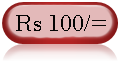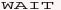Web Development : Starting from Concept, HTML, CSS, Web Site Develpment to Website Launching.
Written by: Sanjay Srivastava
B.Tech (Honours);IIT Kharagpur
PMP ; PMI USA

Class - VIII - Maths:- INDEX

1. Squares & Square Roots
2. Cubes & Cube Roots
4. Profit Loss & Discount
5. Compound Interest
6. Algebraic Identities7. Polynomials8. Linear Equations9. Parallel Lines10. Types of Quadrilaterals11. Construction of Quadrilaterals12. Circles13. Areas14. Surface Areas15. Volumes16. StatisticsClass - VIII - Maths

## How to Calculate Profit and Loss (Page 2/4)

Question - Type 3 -
Gopal purchase 10 chairs @ Rs 100 per chair. He sold 4 chairs @ Rs 120 per chair and 6 chairs @ Rs 95 per chair. What is his profit / loss & what %

C.P. = 10 * 100 = Rs 1000/=
S.P. = 4*120 + 6 * 95 = 480+570 = 1050

Since S.P. is more than C.P. therefore it's is a profit of Rs 1050-1000 = 50/-

profit % = profit*100 / C.P.
= 50 * 100 / 1000= 5

therefore profit % = 5 %

Question - Type 4 -
Raju purchase 4 table @ Rs 100 per table and 4 table @ 110 per table. He sold all table @ 105 per table. What is his profit / loss %